## Monday, August 6, 2007

### Irodov Problem 1.65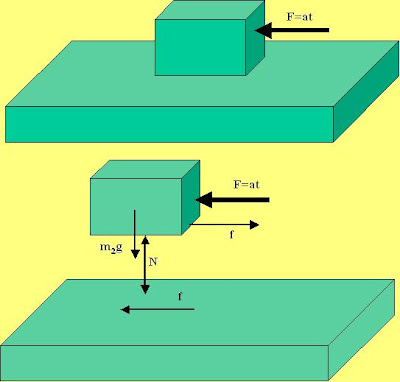The key thing to understand in this problem is that the friction force is responsible for transmitting the force F from the mass to the plank.

Lets first understand what happens in this problem. At first, when the force F is not too large, the friction between the two bodies (the plank and the mass) is sufficient to couple the two bodies together and avoid any slipping between them. Thus, initially the two bodies accelerate together like one body.

However, F increases with time and there will come a time when F is too large for friction between the bodies to maintain the coupling between the bodies and then the mass will start to slip relative to the plank. When this happens, only the friction

Let f be the force of friction acting between the two surfaces at any given time and let N be the
normal reaction acting between then two surfaces.

Dynamics of the mass: There are two forces acting on the mass in the vertical direction, the normal reaction from the plank N and the force of gravity. There is no acceleration for this mass along the vertical direction. Hence,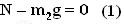Along the horizontal direction there are two forces acting on the mass, the force F and the friction force f that opposes the relative motion between the bodies. Let the acceleration of the mass be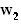then,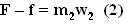Dynamics of the plank : There is only one horizontal force acting on the plank namely the friction force f and this force is solely responsible for accelerating the plank. Let the acceleration of the plank be. Thus, we have,The maximum force of friction that can be produced between the surfaces is given by Nk so the moment this value is reached, the bodies will start to slip relative to each other. Until this value is reached they will move together like one single body. When the bodies are moving together,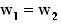. Let this common acceleration be w. From (3) and (2) we have,The force of friction between the bodies during this phase can be determined by (3) as,Using (1), the maximum possible force of friction between the surfaces is given by,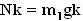Thus, just before the slipping condition,After this time, the bodies will start to slip relative to each other and the value of force of friction between the two bodies will be fixed at,. Thus, now from equations (2) and (3) we have,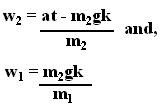Thus, finally we have,Anonymous said...

excellen explanation exhortiveAnonymous said...

smart work man..